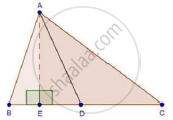Share

# In an Acute-angled Triangle, Express a Median in Terms of Its Sides. - CBSE Class 10 - Mathematics

ConceptApplication of Pythagoras Theorem in Acute Angle and Obtuse Angle

#### Question

In an acute-angled triangle, express a median in terms of its sides.

#### SolutionWe have,

In ΔABC, AD is a median.

Draw AE ⊥ BC

In ΔAEB, by pythagoras theorem

AB2 = AE2 + BE2

⇒ AB2 = AD2 − DE2 + (BD − DE)2            [By Pythagoras theorem]

⇒ AB2 = AD2 − DE2 + BD2 + DE2 − 2BD × DE

⇒ AB2 = AD2 + BD2 − 2BD × DE

⇒ AB2 = AD2 + "BC"^2/4 - BC x DE         ...(i)  [BC = 2BD given]

Again, In ΔAEC, by pythagoras theorem

AC2 = AE2 + EC2

⇒ AC2 = AD2 − DE2 + (DE + CD)2             [By Pythagoras theorem]

⇒AC2 = AD2 + CD2 + 2CD × DE

⇒ AC2 = AD2 + "BC"^2/4 + BC x DE     ...(ii)   [BC = 2CD given]

AB2 + AC2 = 2AD2 + "BC"^2/2

⇒ 2AB2 + 2AC2 = 4AD2 + BC2           [Multiply by 2]

⇒4AD2 = 2AB2 + 2AC2 − BC2

⇒ AD2 = (2"AB"^2+2"AC"^2-"BC"^2)/4

Is there an error in this question or solution?

#### Video TutorialsVIEW ALL 

Solution In an Acute-angled Triangle, Express a Median in Terms of Its Sides. Concept: Application of Pythagoras Theorem in Acute Angle and Obtuse Angle.
S